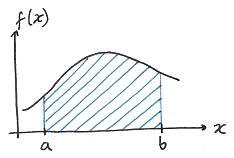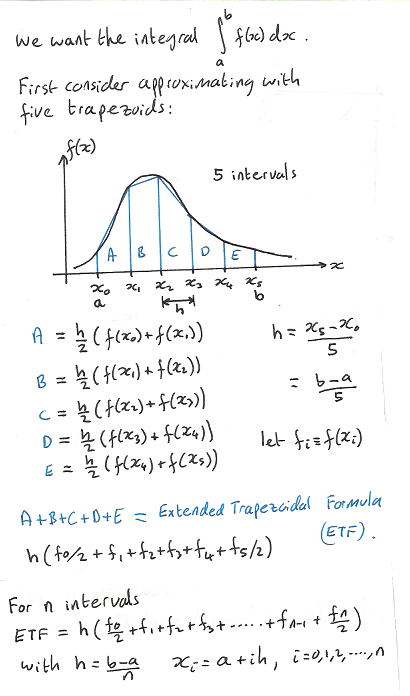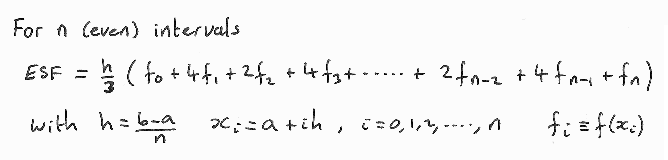http://cpp.gantep.edu.tr
C++ resources. Faculty of Engineering, University of Gaziantep
MAIN  -  Tutorials  -  Howtos  -  nmPrimer  -  Misc  -  Links  -  Contacts

Complete: ##################-- (90%)

Integration of a function f(x)1.   The Extended Trapezoidal Formula (ETF) 2.   The Extended Simpson's Formula (ESF)

The integral of a function f(x) between the limits a and b is simply the area under the curve between a and b. Finding the integral analytically may be difficult and so a numerical solution may be necessary or simply convenient. The following sections describe two methods of numerical integration, they are the most simple cases of the Newton-Cotes formulas which are a group of formulas based on evaluating the integrand at n+1 equally-spaced points.The ETF is implementated in the program below.

nmprimer-ETF.cpp: Extended Trapezoidal Formula for the integral of a function.
 ``` 1: 2: 3: 4: 5: 6: 7: 8: 9: 10: 11: 12: 13: 14: 15: 16: 17: 18: 19: 20: 21: 22: 23: 24: 25: 26: 27: ``` ```// Numerical integration via the Extended Trapezoidal Formula (ETF) #include #include using namespace std; double f(double x) { double y = 1.76 + 3.21*x*x; return 894.*x / (y*y*y); } int main() { const int n=100; const double a=0.0, b=1.61; const double h = (b-a)/n; double etf = (f(a)+f(b))/2; for (int i=1; i

In this program the function f(x) = 894 x / ( 1.76 + 3.21 x2 )3 is integrated over the range x=0 to x=1.61. The solution, to 6 d.p. accuracy is 21.792290; the result from the ETF with n=100 is 21.788672 and so the error is -0.003618 (about 0.02% error). It can be shown that the truncation error in the ETF is proportional to 1/n2. To illustrate this we can re-run the above program with n=1000 (ten times the previous value, the result is 21.792253 and so the error is -0.000037 and so the error is reduce by a factor of 100 = 102 as expected. Note that if we increase the value of n too much then round-off errors will dominate the error. You can investigate round-off and truncation errors by playing with the value of n in the above program.

2.   The Extended Simpson's Formula (ESF)

In the Extended Simspson Formula (ESF) trapezoids are replaced by the quadratic polynomials; the truncation error in the ESF is proportional to 1/n4 and so we should expect results with much greater accuracy compared to the ETF.The ESF is implementated in the program below to solve the same problem as the above ETF program.

nmprimer-ESF.cpp: Extended Simpson's Formula for the integral of a function.
 ``` 1: 2: 3: 4: 5: 6: 7: 8: 9: 10: 11: 12: 13: 14: 15: 16: 17: 18: 19: 20: 21: 22: 23: 24: 25: 26: 27: ``` ```// Numerical integration via the Extended Simpson's Formula (ESF) #include #include using namespace std; double f(double x) { double y = 1.76 + 3.21*x*x; return 894.*x / (y*y*y); } int main() { const int n=100; const double a=0.0, b=1.61; const double h = (b-a)/n; double esf = f(a)+f(b); for (int i=1; i<=n-1; i++) esf += 2.0 * pow(2.0,double(i%2)) * f(a+i*h); esf *= h/3; cout.precision(9); cout << "The integral = " << esf << endl; }``` ```The integral = 21.7922915 ```

The result from the ESF with n=100 is 21.792292 and so the error is 0.000002 which is far more accurate than that obtained by the ETF.

The choice of the value of n in both the ETF and ESF may require some experimentation. Observing the difference between a solution evaluated with n intervals and a solution evaluated with 2n intervals is usually a reasonable estimate of the error in the evaluation with n intervals. Using this idea one can modify the above programs to evaluate the integral with increasing number of intervals until a predetermined tolerance is reached. This is left to the reader as an exercise.

Complete: ##################-- (90%)